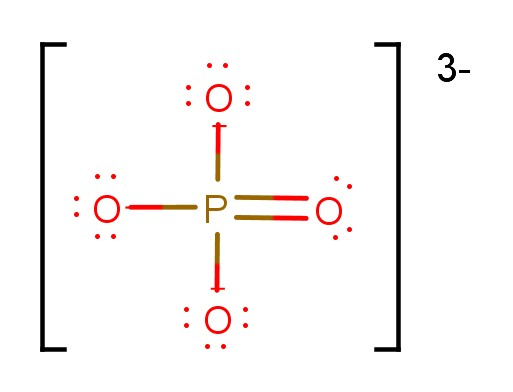# Problem: What is the hybridization of the central atom in each of the following?c. PO43−

###### FREE Expert Solution

Hybridization - depends on # of electron groups around central atom

P (less electronegative than O) → central atom

Total number of valence electrons:

Group            Valence Electrons

P        5A                  1 × 5 e = 5 e

O        6A                 4 × 6 e = 24 e

From -3 charge               Add3 e

Total:  32 valence e

Lewis structure:Orbitals that can be used by the central atom:

Subshell                   Total # of orbitals

s           _                1 orbital

p          _ _ _             3 orbitals

d        _ _ _ _ _          5 orbitals

There are 4 groups around central element:

▪ 4 O atoms + 0 lone pairs = 4 groups (needs 4 orbitals)

88% (19 ratings)###### Problem Details

What is the hybridization of the central atom in each of the following?

c. PO43−

Frequently Asked Questions

What scientific concept do you need to know in order to solve this problem?

Our tutors have indicated that to solve this problem you will need to apply the Hybridization concept. You can view video lessons to learn Hybridization. Or if you need more Hybridization practice, you can also practice Hybridization practice problems.

What professor is this problem relevant for?

Based on our data, we think this problem is relevant for Professor Bellert's class at BAYLOR.

What textbook is this problem found in?

Our data indicates that this problem or a close variation was asked in Chemistry - OpenStax 2015th Edition. You can also practice Chemistry - OpenStax 2015th Edition practice problems.